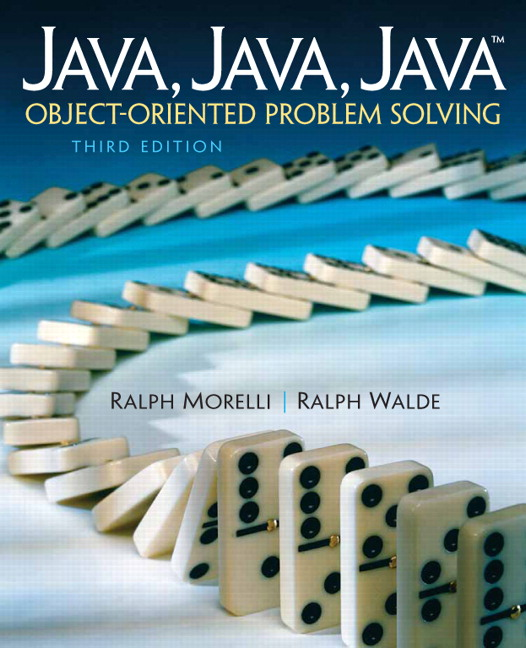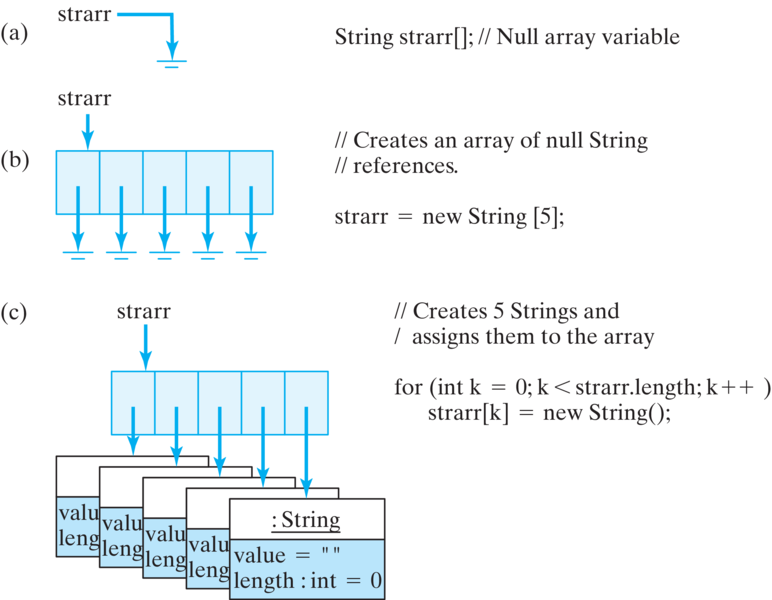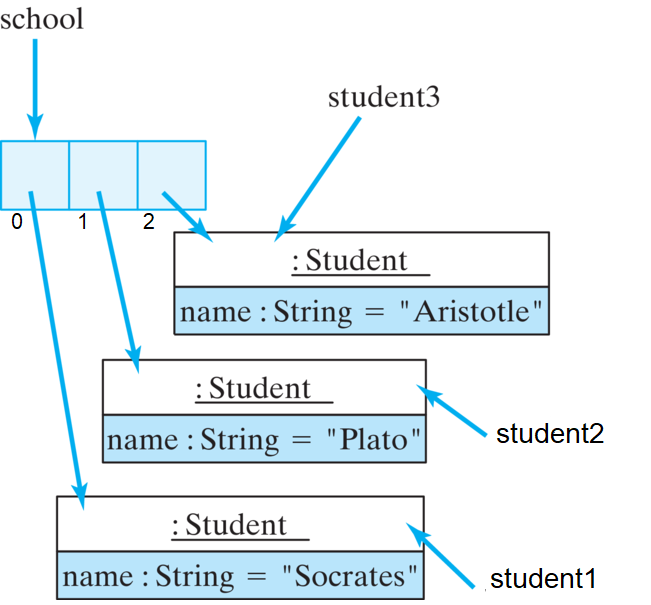# Java, Java, Java: Object-Oriented Problem Solving, 2022E

## Section9.2One-Dimensional Arrays

An array is considered a data structure, which is an organized collection of data. In an array, data are arranged in a linear or sequential structure, with one element following another. When referencing elements in an array, we refer to the position of the particular element within the array.

### Subsection9.2.1Array Subscripts/Indexing

For example, if the array is named arr, then the elements are named arr, arr, arr, ... arr[n-1], where n gives the number of elements in the array. This naming also reflects the fact that the array’s data are contained in storage locations that are next to each other. In Java, as in C, C++, and some other programming languages, the first element of an array has index 0. (This is the same convention we used for String s.)
Figure 9.2.1 shows an array named arr that contains 15 int elements. The syntax for referring to elements of an array is
 arrayname[ subscript ]
where arrayname is the name of the array — any valid identifier will do — and subscript, also called index, is the position of the element within the array. As Figure 9.2.1 shows, the first element in the array has subscript 0, the second has subscript 1, and so on.
A subscript or index is an integer quantity contained in square brackets that is used to identify an array element. A subscript must be either an integer value or an integer expression, for example arr[j+k] where j+k is evaluated to an integer before being used to pull out the array element. Try the code below which uses Figure 9.2.1 as an example. suppose that j and k are integer variables equaling 5 and 7, respectively. Each of the following then would be valid references to elements of the array arr.

#### Activity9.2.1.

Run the following code. Can you print out the 0th element of the array? Can you print out the (k-j)th element? Which element is that?
It is a syntax error to use a non-integer type as an array subscript. Each of the following expressions would be invalid (try them above to see what happens):
arr[5.0]  // 5.0 is a float and can't be an array subscript
arr["5"]  // "5" is a string not an integer

For a given array, a valid array subscript must be in the range 0 ... N-1, where N is the number of elements in the array or it is considered out-of-bounds. An out-of-bounds subscript creates a run-time error — that is, an error that occurs when the program is running — rather than a syntax error, which can be detected when the program is compiled. For the array arr, each of the following expressions contain out-of-bounds subscripts:
arr[-1]  // Arrays cannot have negative subscripts
arr['5'] // Char '5' promoted to its Unicode value, 53
arr  // The last element of arr has subscript 14
arr[j*k] // Since j*k equals 35

Each of these references would lead to an IndexOutOfBoundsException. Try them above to see this exception. (Exceptions are covered in detail in Chapter 10.)

### Subsection9.2.2Declaring and Creating Arrays

For the most part, arrays in Java are treated as objects. Like objects, they are instantiated with the new operator and they have instance variables (for example, length). Like variables for objects, array variables are considered reference variables. When arrays are used as parameters, a reference to the array is passed rather than a copy of the entire array.
The primary difference between arrays and full-fledged objects is that arrays aren’t defined in terms of an Array class. Thus, arrays don’t fit into Java’s Object hierarchy. They don’t inherit any properties from Object and they cannot be subclassed.
You can think of an array as a container that contains a number of variables. As we’ve seen, the variables contained in an array object are not referenced by name but by their relative position in the array. The variables are called components. If an array object has N components, then we say that the array length is N. Each of the components of the array has the same type, which is called the array’s component type. An empty array is one that contains zero variables.
A one-dimensional array has components that are called the array’s elements . Their type is the array’s element type. An array’s elements may be of any type, including primitive and reference types. This means you can have arrays of int, char, boolean, String, Object, Image, TextField, TwoPlayerGame and so on.
When declaring a one-dimensional array, you have to indicate both the array’s element type and its length. Just as in declaring and creating other kinds of objects, creating an array object requires that we create both a name for the array and then the array itself. The following statements create the array shown in Figure 9.2.1:
int arr[];          // Declare a name for the array
arr = new int;  // Create the array itself

These two steps can be combined into a single statement as follows:
int arr[] = new int;

In this example, the array’s element type is int and its length is 15, which is fixed and cannot be changed. This means that the array contains 15 variables of type int, which will be referred to as arr, arr, arr.

### Subsection9.2.3Array Allocation

Creating the array in Figure 9.2.1 means allocating 15 storage locations that can store integers. Note that one difference between declaring an array and declaring some other kind of object is that square brackets, [], are used to declare an array type. The brackets can be attached either to the array’s name or to its type, as in the following examples:
int arr[];     // The brackets may follow the array's name
int[] arr;     // The brackets may follow the array's type

The following example creates an array of five Strings and then uses a for loop to assign the strings "hello1", "hello2", "hello3", "hello4", and "hello5" to the five array locations:
String strarr[];                // Declare a name for the array
strarr = new String;              // Create the array itself
// Assign strings to the array
for (int k = 0; k < strarr.length; k++)     // For each element
strarr[k] = new String("hello" + (k + 1)); // Assign a string

Note that the expression k < strarr.length specifies the loop bound. Every array has a length instance variable, which refers to the number of elements contained in the array. As we mentioned, arrays, like String s, are zero indexed, so the last element of the array is always given by its length-1. However, length is an instance variable for arrays, whereas length() is an instance method for String s. Therefore, it would be a syntax error in this example to refer to strarr.length().
In the example, we first use the new operator to create strarr, an array of type String of length five. We then use a String constructor to create the five Strings that are stored in the array. It is important to realize that creating an array to store five Object s (as opposed to five primitive data elements) does not also create the Objects themselves that will be stored in the array.
When an array of objects is created, the array’s elements are references to those objects (Fig. Figure 9.2.5). Their initial values, like all reference variables, are null. So to create and initialize the array strarr, we need to create six objects — the array itself, which will contain five String s, and then the five Strings that are stored in strarr.Figure 9.2.5. Creating an array of five Strings involves six objects, because the array itself is a separate object. In (a), the array variable is declared. In (b), the array is instantiated, creating an array of five null references. In (c), the five Strings are created and assigned to the array.
One more example will help underscore this point. The following statements create four newObjects, an array to store three Students plus the three Students themselves (Figure 9.2.6):
Student school[] = new Student;   // A 3 Student array
school = new Student("Socrates"); // The first Student
school = new Student("Plato");    // The second Student
school = new Student("Aristotle");// The third Student

The first statement creates an array named school to store three Students, and the next three statements create the individual Students and assign them to the array (Figure 9.2.6). Thus, creating the array and initializing its elements require four new statements.
The following sequence of statements would lead to a null pointer exception because the array’s elements have not been instantiated:
Student students[] = new Student; // A 3 Student array
System.out.println(students.getName());

In this case, students is a null reference, thus causing the exception.
Now that we’ve assigned the three Students to the array, we can refer to them by means of subscripted references. A reference to the Student named “Socrates” is now school, and a reference to the Student named “Plato” is school. In other words, to refer to the three individual students we must refer to their locations within school. Of course, we can also use variables, such as loop counters, to refer to a Student’s location within school. The following for loop invokes each Student’s getState() method to print out its current state:
for (int k = 0; k < school.length; k++)
System.out.println(school[k].getState());

What if the three Students already existed before the array was created? In that case, we could just assign their references to the array elements, as in the following example (Figure 9.2.8):
Student student1 = new Student("Socrates");
Student student2 = new Student("Plato");
Student student3 = new Student("Aristotle");
Student school = new Student; // A 3 Student array
school = student1;
school = student2;
school = student3;

In this case, each of the three Student objects can be referenced by two different references — its variable identifier (such as student1) and its array location (such as school).Figure 9.2.8. Arrays of objects store references to the objects, not the objects themselves.
For arrays of objects, Java stores just the reference to the object in the array itself, rather than the entire object. This conserves memory, since references require only 4 bytes each whereas each object may require hundreds of bytes (Figure 9.2.8).
When an array of N elements is created, the compiler allocates storage for N variables of the element’s type. In the case of arr that we discussed earlier (Figure 9.2.1), the compiler would allocate storage for 15 ints —i.e., 60 contiguous bytes of storage, because each int requires 4 bytes (32 bits) of storage. If we declare an array of 20 double s,
double arr[] = new double;

the compiler will allocate 160 bytes of storage — i.e., 20 variables of 8 bytes (64 bits) each. In the case of the Student examples and String examples, because these are objects (not primitive types), the compiler will allocate space for N addresses, where N is the length of the array and where each address requires 4 bytes.

#### ExercisesSelf-Study Exercises

How much memory space (in bytes) would be allocated for each of the following array declarations?
##### 1.How many bytes int array?
int a[] = new int;

##### 2.How many bytes double array?
double b[] = new double;

##### 3.How many bytes char array?
char c[] = new char;

##### 4.How many bytes String array?
String s[] = new String;

##### 5.How many bytes Student array?
Student p[] = new Student;


### Subsection9.2.4Initializing Arrays

Array elements are automatically initialized to default values that depend on the element type: boolean elements are initialized to false, and integer and real types are initialized to 0. Reference types—that is, arrays of objects—are initialized to null.
Arrays can also be assigned initial values when they are created, although this is feasible only for relatively small arrays. An array initializer is written as a list of expressions separated by commas and enclosed by curly braces, { }. For example, we can declare and initialize the array shown in Figure 9.2.1 with the following statement:
int arr[] = {-2,8,-1,-3,16,20,25,16,16,8,18,19,45,21,-2};

Similarly, to create and initialize an array of Strings, we can use the following statement:
String strings[] = {"hello", "world", "goodbye", "love"};

This example creates and stores four Strings in the array. Note in these examples that when an array declaration contains an initializer, it is not necessary to use new and it is not necessary to specify the number of elements in the array. The number of elements is determined from the number of values in the initializer list.

### Subsection9.2.5Assigning and Using Array Values

Array elements can be used in the same way as other variables. The only difference, of course, is that references to the elements are subscripted. For example, the following assignment statements assign values to the elements of two arrays, named arr and strings:
arr = 5;
arr = 10;
arr = 3;
strings = "who";
strings = "what";
strings = strings = "where";

The following loop assigns the first 15 squares —1, 4, 9 ... — to the array arr:
for (int k = 0; k < arr.length; k++)
arr[k] = (k+1) * (k+1);

The following loop prints the values of the array arr:
for (int k = 0; k < arr.length; k++)
System.out.println(arr[k]);


#### ExercisesSelf-Study Exercises

##### 1.What’s the value?
For the following array declaration:
double farr[] = {1.1, 2.2, 3.3, 4.4, 5.5};

what is the value of farr?
##### 2.What’s the value?
For the following array declaration:
double farr[] = {1.1, 2.2, 3.3, 4.4, 5.5};

what is the value of farr[farr.length - 2]?
Hint.
Hint: farr.length equals 5.
##### 3.Access the last element.
For the following variable declarations:
int k = 0;
double farr[] = {1.1, 2.2, 3.3, 4.4, 5.5};

which of the following expressions would correctly access the last element in farr?
• farr
• That’s one way to access the last element.
• farr[farr.length-1]
• That’s one way to access the last element.
• farr[length]
• This expression contains a syntax error: no such variable length.
• farr[k+4]
• That’s one way to access the last element.
##### 4.Assign to the first element.
For the following variable declarations:
int k = 0;
double farr[] = {1.1, 2.2, 3.3, 4.4, 5.5};

which of the following expressions would correctly assing 10.5 to the first element in farr?
• farr(0) = 10.5;
• Nope, we don’t use parentheses.
• farr = 10.5;
• No, don’t forget that arrays are zero-indexed.
• farr[k+1] = 10.5;
• This would assign the value to the second element.
• farr[k] = 10.5;
• Correct. This is equivalent to farr = 10.5;